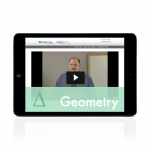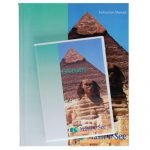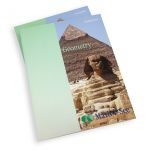## Geometry ProductsIncludes everything you need for a successful experience with Geometry:

• Instruction Manual with complete solutions
• Instruction DVD
• Student Workbook
• Tests booklet

Order HereThe Geometry Student Pack contains the Student Workbook with lesson-by-lesson worksheets, review pages, and honors pages. It also includes the Geometry Tests.s.

Order HereThe Geometry Instruction Pack contains the instruction manual with lesson-by-lesson instructions and detailed solutions, and the DVD with lesson-by-lesson video instruction..

Order HereLifetime access to Geometry streaming instruction videos and online Instruction Manual, lesson and test solutions, and other online resources from any browser. Access begins at time of online purchase or redemption of access code. Note: Digital Packs do not include Student Workbook or Tests.

Order Here

# Geometry

## Points, lines, planes, angles, circles, triangles, quadrilaterals, Pythagorean Theorem, conic sections, proofs and more.

Major Concepts and Skills Include:

• Describing points, lines, rays, line segments, angles, and planes
• Calculating the measure of the interior and exterior angles of a regular polygon
• Understanding the geometry of a circle, sphere, and ellipse
• Understanding and computing volume and surface area of solids
• Using the Pythagorean theorem to identify triangle attributes
• Applying postulates, theorems, definitions, and properties to geometric proofs

• Using a protractor to construct angles
• Using a compass to construct bisectors
• Constructing and identifying triangles
• Working with algebraic expressions containing radicals
• Completing geometric transformations within a Cartesian plane
• Understanding basic trigonometric functions

## Sample Lesson

• Points, Lines, Rays and Line Segments
• Planes and Sets
• Angles
• Types of Angles
• Parallel and Perpendicular Lines with Midpoints and Bisectors
• Supplementary and Complementary Angles
• Transversals with Interior and Exterior Angles
• Perimeter of a Rectangle, Triangle, Parallelogram, and Trapezoid
• Area of a Rectangle, Triangle, Parallelogram, and Trapezoid
• Constructing and Identifying Triangles
• Regular Polygons
• Geometry of a Circle, Sphere and Ellipse
• Inscribed and Circumscribed Figures
• Area and Circumference of a Circle
• Area of an Ellipse
• Latitude and Longitude
• Volume of Rectangular Solid and Cylinder
• Volume of Pyramid, Cone, Prism, and Sphere
• Surface Area of Solids
• Pythagorean Theorem
• Special Triangles: (45°-45°-90°)
• Special Triangles: (30°-60°-90°)
• Axioms and Postulates
• Corresponding Parts of Triangles and Remote Interior Angles
• Proving Triangles Congruent with SSS and SAS
• Proving Triangles Congruent with ASA and AAS
• Proving Triangles Congruent with HL, LL, HA, and LA
• Proving Triangles Similar with AA and Proportion or Ratio
• Transformational Geometry
• Trigonometric Functions: Sine, Cosine and Tangent
• Inverse Trigonometric Functions: Secant, Cosecant, and Cotangent
• Sin² + Cos² = 1

## View Geometry Sample Lesson PDFGeometry Digital Pack - Canadian EditionLifetime access to Geometry streaming instruction videos and online Instruction Manual, lesson and test solutions, and other online resources from any browser. Access begins at time of online purchase or redemption of access code. Note: Digital Packs do not include Student Workbook or Tests.\$64.75Geometry Instruction Pack - Canadian EditionThe Geometry Instruction Pack contains the instruction manual with lesson-by-lesson instructions and detailed solutions, and the DVD with lesson-by-lesson video instruction.\$98.00Geometry Student Pack - Canadian EditionThe Geometry Student Pack contains the Student Workbook with lesson-by-lesson worksheets, review pages, and honors pages. It also includes the GeometryTests.\$63.50Geometry Universal Set - Canadian EditionIncludes everything you need for a successful experience with Geometry: